# Math2.org Math Tables:(Math)

 An Introduction to  Generalized Calculus Unit I - Product Calculus A. Introduction

# I. Product Calculus

## A. Introduction

We will examine our first non-elementary calculus, the product calculus. The product calculus is defined by a whole new set of building blocks. It gets its name from the product expansion,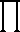, which we will refer to as the discrete product for classification reasons. Unlike the summation,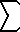, which is the sum of all terms, theis the product of all its terms.

One may propose that we replace thein the discrete iteration block with theand build a new calculus from there:

 Product Calculus Continuous Discrete Limit ? Iteration ?discrete product
The other building blocks must be redefined in order to satisfy the conditions mentioned in the preface:

• The continuous iteration is discrete iteration made continuous.
• The continuous limit and continuous iteration are inverses of each other.
The existence of the other two building blocks is hinted by the following analogy:is toasis to ?

Furthermore, we may ask:is to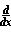asis to ?

In the following sections, we will find out exactly what these building blocks are:
 Product Calculus Continuous Discrete Limit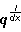antiproduct Iteration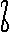continuous productdiscrete product
And from there precipitates a whole new calculus with its own rules and theorems. Yet, it maintains key relationships with elementary calculus.